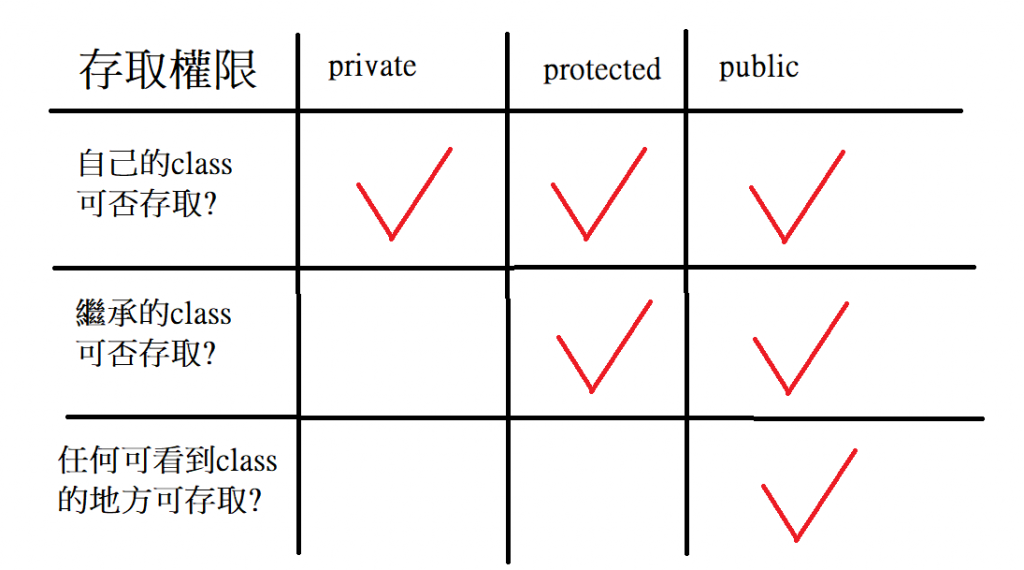#1

## 【c/c++學習筆記】類別中的存取權限: public, private, protected

• public猶如你在iT邦幫忙發技術文章，人人可以看到
• private猶如你不想給人看到的秘密日記，只有自己可以看
• protected雖是隱私的訊息，但跟親近的人分享還是可以的，譬如你朋友或你小孩`secret``cook`兩種方法

`size`屬性和`cook`方法定義成`public`

``````class ElectricPot
{
private:
string color;
void secret(){
std::cout << "神秘功能"<<std::endl;
}
public:
int size;
void cook(){
std::cout << "我是"<< this->color <<"顏色的電鍋，我正在煮飯"<<std::endl;
}
};
``````

``````class ElectricPot
{
string color;
void secret(){
std::cout << "神秘功能"<<std::endl;
}
public:
int size;
void cook(){
std::cout << "我是"<< this->color <<"顏色的電鍋，我正在煮飯"<<std::endl;
}
};
``````

## 合法的存取

``````int main()
{
ElectricPot myPot;
myPot.size = 20;
myPot.cook();
}
``````

## 不合法的存取

``````int main()
{
ElectricPot myPot;
myPot.size = 20;
myPot.cook();
std::cout << myPot.color << std::endl; //錯誤: color是private成員
myPot.color = "yellow"; //錯誤: color是private成員
myPot.secret(); //錯誤: secret()是private成員
return 0;
}
``````

# 完整的範例程式

``````#include <iostream>
#include <string>
using std::string;

class ElectricPot
{

string color;
void secret() {
std::cout << "神秘功能" << std::endl;
};
public:
int size;
void cook() {
std::cout << "我是" << this->color << "顏色的電鍋，我正在煮飯" << std::endl;
}
};

int main()
{
ElectricPot myPot;
myPot.size = 20;
myPot.cook();
return 0;
}
``````

# 什麼時候用private?

class能做的事情則定義為`public`讓其它地方可以使用。

``````class Rectangle
{
int x, y;
public:
void set_values(int x, int y) {
this->x = x;
this->y = y;
};
};
``````

``````Rectangle r;
r.set_values(3,4); //合法
r.x = 3; //不合法，因為x是private
``````